# Platonic solids

Keywords: Platonic solids, also called the regular solids or regular polyhedra
Name
Tetrahedron
Hexahedron
(Cube)
Octahedron
Dodecahedron
Icosahedron
Picture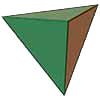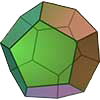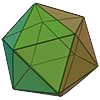Net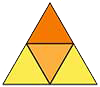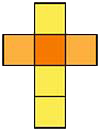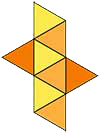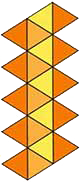Faces
4
6
8
12
20
Face type
equilateral triangle
square
equilateral triangle
equilateral pentagon
equilateral triangle
Duality
tetrahedron
octahedron
hexahedron
icosahedron
dodecahedron
Edges
6
12
12
30
30
Vertices
4
8
6
20
12
Edges from vertex
3
3
4
3
5
Number of diagonals
0
4
3
100
36
Dihedral angle
≈70°31'43,61''
90°
≈109°28'16,39''
≈116°33'55,84''
≈138°11'22,87''
Surface area
$\sqrt{3}{a}^{2}$
$6{a}^{2}$
$2\sqrt{3}{a}^{2}$
$3\sqrt{25+10\sqrt{5}}\phantom{\rule{thickmathspace}{0ex}}{a}^{2}$
$5\sqrt{3}{a}^{2}$
Volume
$\frac{\sqrt{2}{a}^{3}}{12}$
${a}^{3}$
$\frac{\sqrt{2}{a}^{3}}{3}$
$\frac{\left(15+7\sqrt{5}\right){a}^{3}}{4}$
$\frac{\left(15+5\sqrt{5}\right){a}^{3}}{12}$
$\frac{\sqrt{6}a}{4}$
$\frac{\sqrt{3}a}{2}$
$\frac{\sqrt{3}a}{2}$
$\frac{\sqrt{3}\left(1+\sqrt{5}\right)a}{4}$
$\frac{\sqrt{10+2\sqrt{5}}\phantom{\rule{thickmathspace}{0ex}}a}{4}$
$\frac{\sqrt{6}a}{12}$
$\frac{a}{2}$
$\frac{\sqrt{6}a}{6}$
$\frac{1}{2}\sqrt{\frac{25+11\sqrt{5}}{10}}\phantom{\rule{thickmathspace}{0ex}}a$
$\frac{\sqrt{42+18\sqrt{5}}}{12}\phantom{\rule{thickmathspace}{0ex}}a$
$\frac{\sqrt{2}a}{4}$
$\frac{\sqrt{2}a}{2}$
$\frac{a}{2}$
$\frac{\left(\sqrt{5}+3\right)a}{4}$
$\frac{\left(1+\sqrt{5}\right)a}{4}$